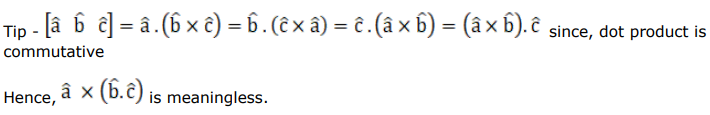# Mark against the correct answer in each of the following:

Question:

Mark $(\sqrt{)}$ against the correct answer in each of the following:

Which of the following is meaningless?

A. $\vec{a} \cdot(\vec{b} \times \vec{c})$

B. $\overrightarrow{\mathrm{a}} \times(\overrightarrow{\mathrm{b}} \cdot \overrightarrow{\mathrm{c}})$

C. $(\overrightarrow{\mathrm{a}} \times \overrightarrow{\mathrm{b}}) \cdot \overrightarrow{\mathrm{c}}$

D. none of these

Solution: